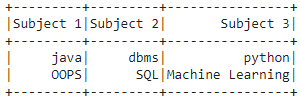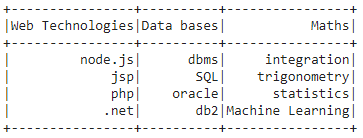PySpark – Create DataFrame from List

• Last Updated : 30 May, 2021

In this article, we are going to discuss how to create a Pyspark dataframe from a list.

To do this first create a list of data and a list of column names. Then pass this zipped data to spark.createDataFrame() method. This method is used to create DataFrame. The data attribute will be the list of data and the columns attribute will be the list of names.

dataframe = spark.createDataFrame(data, columns)

Example1: Python code to create Pyspark student dataframe from two lists.

Python3

 # importing moduleimport pyspark  # importing sparksession from # pyspark.sql modulefrom pyspark.sql import SparkSession  # creating sparksession and giving # an app namespark = SparkSession.builder.appName('sparkdf').getOrCreate()  # list  of college data with two listsdata = [["java", "dbms", "python"],         ["OOPS", "SQL", "Machine Learning"]]  # giving column names of dataframecolumns = ["Subject 1", "Subject 2", "Subject 3"]  # creating a dataframedataframe = spark.createDataFrame(data, columns)  # show data framedataframe.show()

Output:Example 2: Create a dataframe from 4 lists

Python3

 # importing moduleimport pyspark  # importing sparksession from # pyspark.sql modulefrom pyspark.sql import SparkSession  # creating sparksession and giving # an app namespark = SparkSession.builder.appName('sparkdf').getOrCreate()  # list  of college data with two listsdata = [["node.js", "dbms", "integration"],        ["jsp", "SQL", "trigonometry"],        ["php", "oracle", "statistics"],        [".net", "db2", "Machine Learning"]]  # giving column names of dataframecolumns = ["Web Technologies", "Data bases", "Maths"]  # creating a dataframedataframe = spark.createDataFrame(data, columns)  # show data framedataframe.show()

Output:Attention geek! Strengthen your foundations with the Python Programming Foundation Course and learn the basics.

To begin with, your interview preparations Enhance your Data Structures concepts with the Python DS Course. And to begin with your Machine Learning Journey, join the Machine Learning - Basic Level Course

My Personal Notes arrow_drop_up